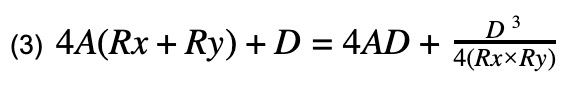Stableswap Invariant Pools
There are some situations where the xy=k formula may not be the best choice to provide the best possible execution for traders. For example, this is the case for pairs of tokens with an exchange rate that rarely deviates from 1:1.
In cases like these, stableswap AMMs are much more capital-efficient than their constant product counterparts, since most of this amplified liquidity is used to facilitate trades around the 1:1 target exchange rate.

## How Stableswap Invariant Pools Work

Stableswap pools are the middle ground between constant product pools and constant price pools. A constant price pool is similar to a constant product pool, but uses a constant sum formula Rx+Ry=k, resulting in a constant price ∆x / ∆y = 1.
The stableswap invariant algorithm uses an amplification parameter A that determines how close the stableswap curve should be to the constant product curve; an amplification value of 0 (A = 0) achieves results identical to the constant product pool algorithm; higher values of A push the curve closer to the constant price curve, resulting in lower slippage for exchange rates close to 1:1.
The formula for the stableswap invariant works by combining terms representing the constant product and constant sum formulas. It's using the constant D to represent the total number of tokens in the pool when token X and token Y have an equal price, and A represents the amplification parameter. The formula can be written as: